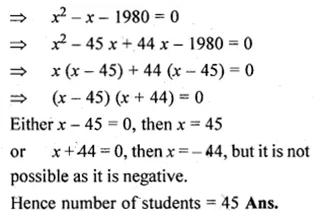Guru

# At an annual function of a school, each student gives the gift to every other student. If the number of gifts is 1980, find the number of students.

• 0

This question has been taken from Book:- ML aggarwal, Avichal publication, class10th, quadratic equation in one variable, chapter 5, exercise 5.5
This is an important ques

At an annual function of a school, each student gives the gift to every other student. If the number of gifts is 1980,find the number of students.

Question no. 24 , ML Aggarwal, chapter 5, exercise 5.5, quadratic equation in one variable, ICSE board,

Share